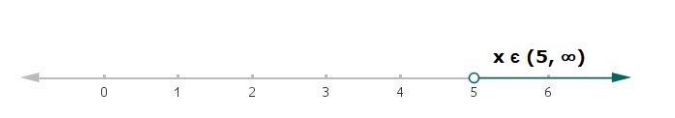# Solve each of the following in equations and represent the solution set on

Question:

Solve each of the following in equations and represent the solution set on the number line.

$3 x-4>x+6$, where $x \in R$

Solution:

Given:

$3 x-4>x+6$, where $x \in R$

$3 x-4>x+6$

Adding 4 to both sides in above equation

$3 x-4+4>x+6+4$

$3 x>x+10$

Now, subtracting x from both the sides in above equation

$3 x-x>x+10-x$

$2 x>10$

Now, dividing both the sides by 2 in above equation

$\frac{2 x}{2}>\frac{10}{2}$

$x>5$

Therefore, $x \in(5, \infty)$